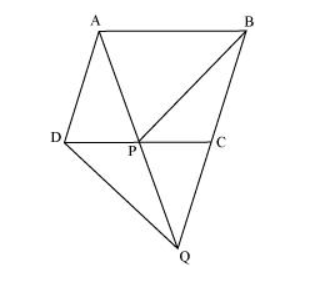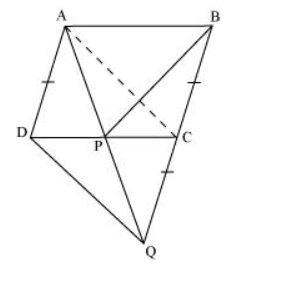# In the following figure, ABCD is parallelogram and BC is produced to a point Q such that AD = CQ.

Question. In the following figure, ABCD is parallelogram and BC is produced to a point Q such that AD = CQ. If AQ intersect DC at P, show that ar (BPC) = ar (DPQ).Solution:

It is given that ABCD is a parallelogram.

AD || BC and AB || DC(Opposite sides of a parallelogram are parallel to each other)

Join point A to point C.Consider $\triangle A P C$ and $\triangle B P C$

$\triangle A P C$ and $\triangle B P C$ are lying on the same base $P C$ and between the same parallels $P C$ and $A B$. Therefore,

Area $(\Delta \mathrm{APC})=$ Area $(\Delta \mathrm{BPC}) \ldots(1)$

In quadrilateral ACDQ, it is given that

$A D=C Q$

Since $A B C D$ is a parallelogram,

$A D \| B C$ (Opposite sides of a parallelogram are parallel)

$C Q$ is a line segment which is obtained when line segment $B C$ is produced.

$\therefore A D \| C Q$

We have,

$A C=D Q$ and $A C \| D Q$

Hence, $A C Q D$ is a parallelogram.

Consider $\triangle \mathrm{DCQ}$ and $\triangle \mathrm{ACQ}$

These are on the same base $C Q$ and between the same parallels $C Q$ and $A D$. Therefore,

Area $(\Delta D C Q)=$ Area $(\triangle A C Q)$

$\Rightarrow$ Area $(\Delta \mathrm{DCQ})$ - Area $(\Delta \mathrm{PQC})=$ Area $(\Delta \mathrm{ACQ})$ - Area $(\Delta \mathrm{PQC})$

$\Rightarrow$ Area $(\Delta \mathrm{DPQ})=$ Area $(\triangle \mathrm{APC}) \ldots(2)$

From equations (1) and (2), we obtain

Area $(\Delta B P C)=$ Area $(\Delta D P Q)$